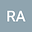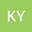2D Crank-Nicolson method for Cahn-Hilliard model of phase separation with a logarithmic free energy
••• Reza Abazari,
• Kenan Yildirim
Reza Abazari
university of Mohaghegh Ardabili
Author ProfileKenan Yildirim
Mus Alparslan Universitesi
Author Profile#### Peer review status:UNDER REVIEW

02 Apr 2020Submitted to Mathematical Methods in the Applied Sciences
04 Apr 2020Assigned to Editor
04 Apr 2020Submission Checks Completed
05 Apr 2020Reviewer(s) Assigned

## Abstract

The mathematical modeling of phase separation of a binary alloy is formulated by a nonlinear evolution equation, known as Cahn-Hilliard equation with various free energy function. The Cahn--Hilliard equation generaly is a forth--order nonlinear equation and its analytical solution does not exist except trivial solutions. In this paper, a second--order splitting finite difference scheme, based on 2D Crank--Nicolson method, is investigated to approximate the solution of the 2D Cahn-Hilliard equation with a logarithmic free energy under homogeneous boundary conditions. We have proved that the scheme has a unique solution and we have studied error estimation for the numerical scheme. Also, we have shown that the proposed method preserves the conservation of mass and the decrease of the total energy. Finally, two numerical experiments are carried out to test the performance of proposed scheme.